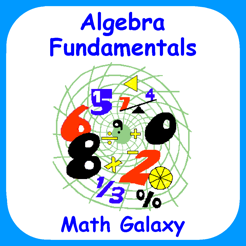## Screenshots

•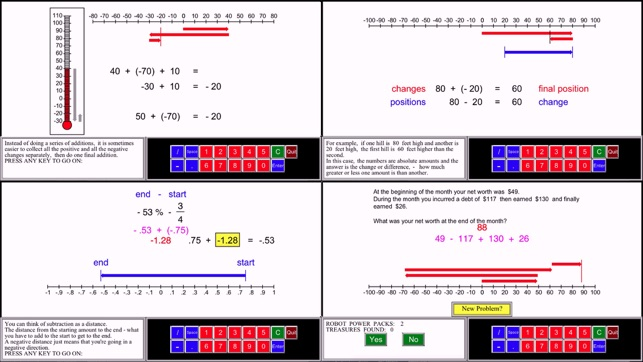•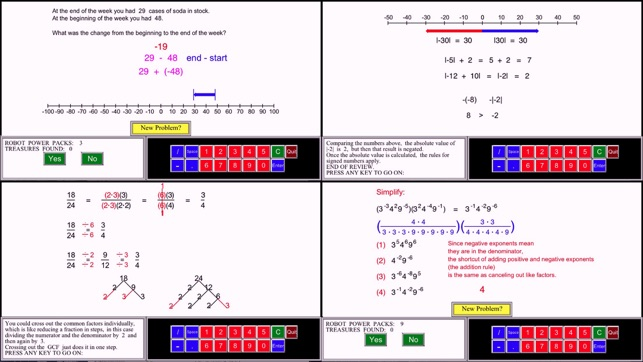•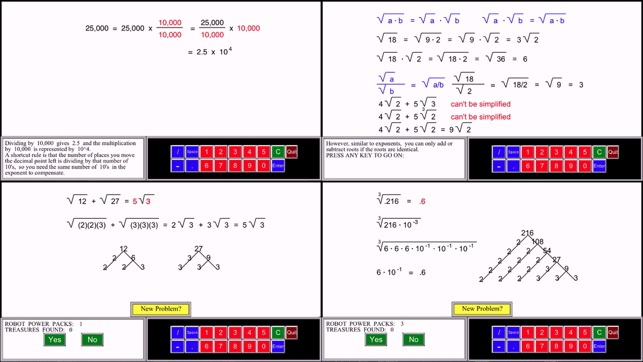•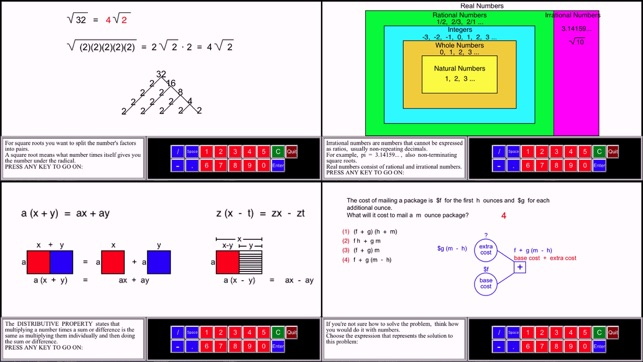•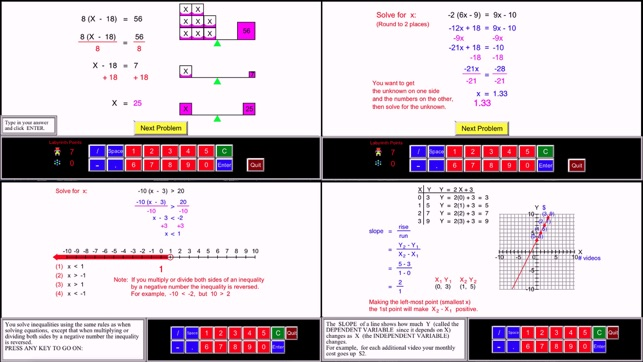•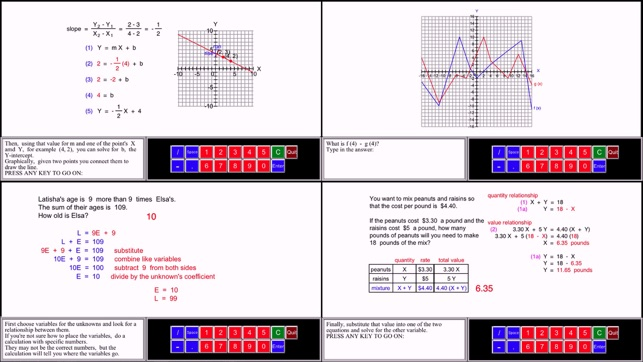•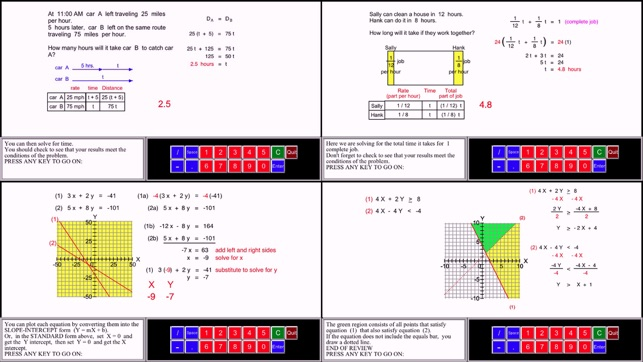•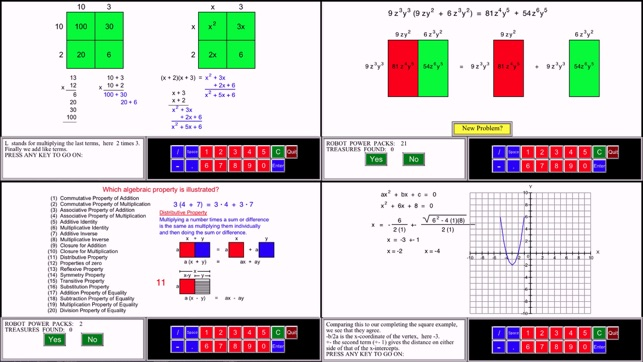•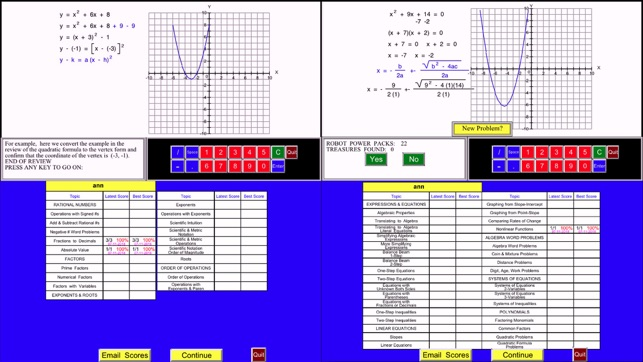•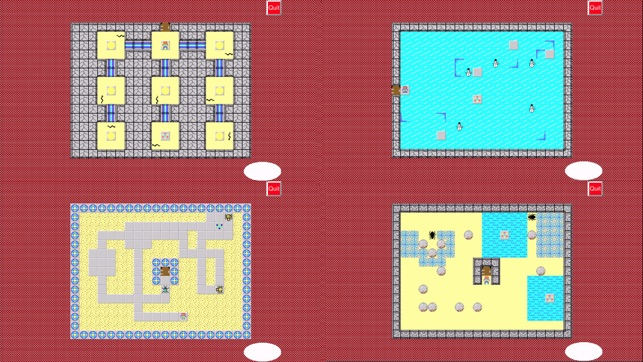## Description

"The French mathematician Jacques S. Hadamard found, in a study of 100 leading mathematicians [including Einstein], that the majority of them were primarily visual in their approach."

Scientific American, Sept., 1984, p. 56.

Math Galaxy Algebra Fundamentals is like having an interactive textbook, with explanations, practice, and games that cover all topics found in an algebra 1 textbook: rational numbers, absolute value, powers/exponents, scientific and metric notation and operations, order of operations, factors and prime numbers, algebraic properties, translating English to algebra, simplifying expressions, 1 & 2-step equations, solving equations with parentheses, fractions, decimals and unknowns on both sides, slopes and linear equations, algebra word problems, systems of equations, systems of inequalities, and polynomials.

The concepts are presented in bite-size pieces, with detailed explanations, illustrations, and an unlimited number of practice problems, with numbers chosen randomly.
Includes support for Schoolwork.

By solving problems students earn robots to use in a maze game called the Labyrinth.

Topics covered include:

RATIONAL NUMBERS

Review Signed Numbers
Operations with Signed Numbers
Negative Number Word Problems
Converting Fractions to Decimals
Negative Numbers & Absolute Value
Explanation of Prime Numbers
Prime Factors
Review Greatest Common Factor
Numerical Factors
Factors with Variables

EXPONENTS & ROOTS

Review of Exponents
Exponents
Review of Operations with Exponents
Operations with Exponents
Review of Scientific Notation
Scientific Intuition
Scientific & Metric Notation
Review of Scientific Operations
Scientific & Metric Operations
Order of Magnitude
Review of Roots
Roots

EXPRESSIONS & EQUATIONS

Order of Operations
Operations with Exponents & Parentheses
Algebraic Properties
Translating to Algebra
Literal Equations
Simplifying Algebraic Expressions
More Simplifying Algebraic Expressions
The Balance Beam 1-Step
The Balance Beam 2-Step
One-Step Equations
Two-Step Equations
Equations with Unknown on Both Sides
Equations with Parentheses
Equations with Fractions or Decimals
One-Step Inequalities
Two-Step Inequalities

LINEAR EQUATIONS

Review Coordinates & Slopes
Slopes
Review Linear Equations
Linear Equations
Graphing from Slope-Intercept
Graphing from Point-Slope
Comparing Rates of Change
Nonlinear Functions

ALGEBRA WORD PROBLEMS

Algebra Word Problems
Coin & Mixture Problems
Distance Problems
Digit, Age, Work Problems

SYSTEMS OF EQUATIONS

2-Variable Review
3-Variable Review
2-Variable Problems
3-Variable Problems
Systems of Inequalities

POLYNOMIALS

Review Polynomials
Derivation of Parabola’s Vertex
Factoring Monomials
Distributive Property / Common Factors
Rational Equation Problems
Rational Equation Word Problems

## What’s New

Version 2.0

Added Schoolwork support for teachers with Apple School Manager and iOS 11.3 or later.
For exercises done in step-by-step mode it reports time on task and number of problems completed.
In final-answer-only mode it also reports a percentage score.
For other users the exercises are the same and scores are reported in the app or can be emailed.
For other users iOS 8.0 or later is required.

## Information

Seller
Gilbert Reilly
Size
21.4 MB
Category
Education
Compatibility

Requires iOS 8.0 or later. Compatible with iPhone, iPad, and iPod touch.

Languages

English

Age Rating
4+
Price
\$4.99

## Supports

•